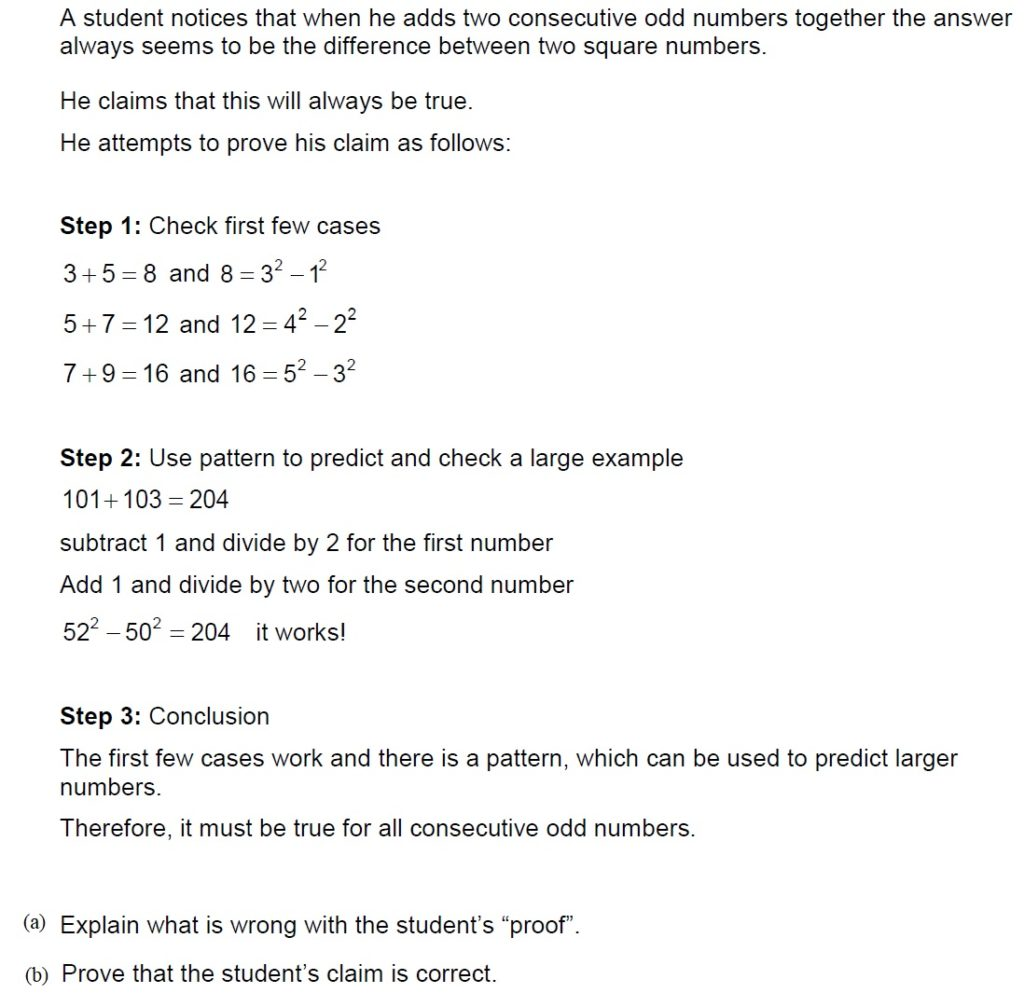# Practice – Reasoning and Proof

1. Show that for all real numbers $a$ and  $b$

$\left|a+b\right|\le \left|a\right|+\left|b\right|$ .       (Triangle Inequality)

2. If  $x$ and  $y$ are not negative real numbers with  $x\le y$ , then  $\sqrt{x}\le \sqrt{y}$ .

3. For any real numbers  $x$ and  $y$ , show that  $\sqrt{{x}^{2}+{y}^{2}}\le \left|x\right|+\left|y\right|$ .

4. Prove that if a, b,  c are positive integers with a2 + b2 = c2 then either a or b is even.

5. Prove that  $\sqrt{3}–\sqrt{2}$ is irrational. (prove by contradiction)

6. Prove that there does not exist a smallest positive real number.

7. If x , y , z are positive real numbers. Prove that x > z and y2 = xz implies that x > y > z.

8. Prove that if $f$ and  $g$ are differentiable functions with $x=f\left(x\right)g\left(x\right)$ , then either $f\left(0\right)\ne 0$ or $g\left(0\right)\ne 0$ .

9. Prove by contradiction that $\sqrt{2}$  is an irrational number.

10. Prove that
n is a prime number greater than 5 $⇒{n}^{4}$ has final digit 1.

11. A student argues that when a rational number is multiplied by an irrational number the result will always be an irrational number.
(a) Identify the rational number for which the student’s argument is not true.

(b) Prove that the student is right for all rational numbers other than the one you have identified in part (a).

12